### Home > ACC6 > Chapter 7 Unit 7 > Lesson CC1: 7.3.2 > Problem7-96

7-96.

On your paper, copy the chart below. Then complete two trials by reading the algebraic expressions. Write in the steps.

Steps

Trial 1

Trial 2

Algebraic Expression

1.

$x$

2.

$6x$

3.

$6x + 24$

4.

$6x + 18$

5.

$x + 3$

6.

$3$

The second row of the table, first column, says: 1. Pick a number.

The third row of the table, first column, now says: 2. Multiply by 6.

The fourth row of the table, first column now says: 3. Add 24.

The fifth row of the table, first column now says: 4. Subtract 6.

The sixth row of the table, first column now says: 5. Divide by 6.

The seventh row of the table, first column now says: 6. Subtract original number.﻿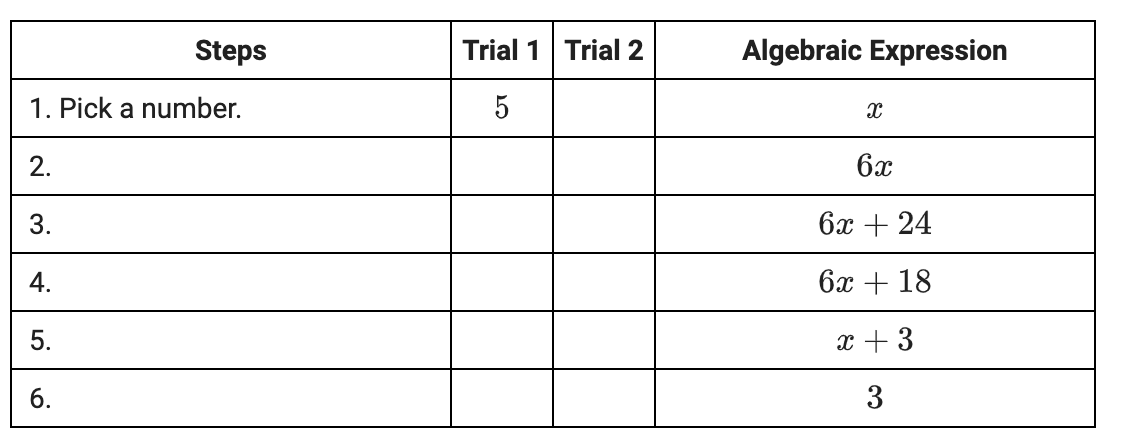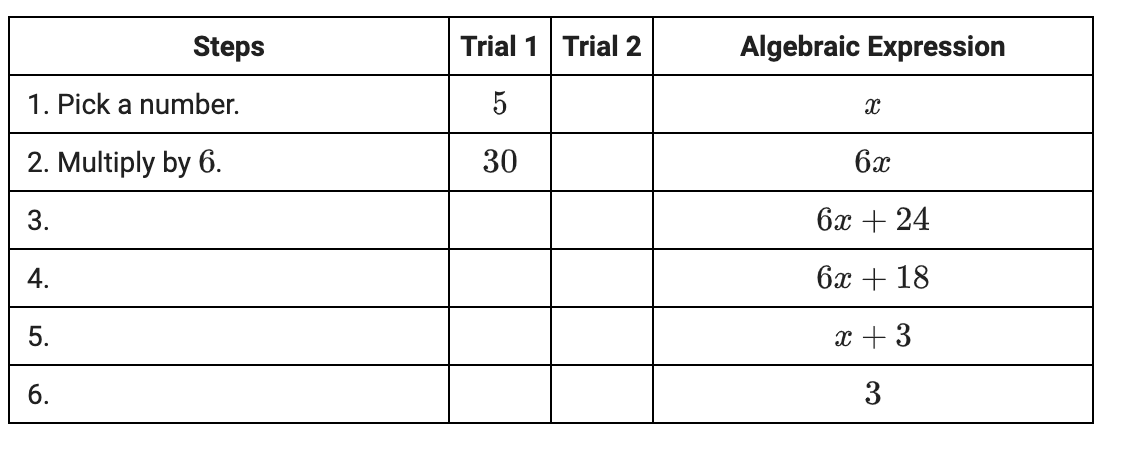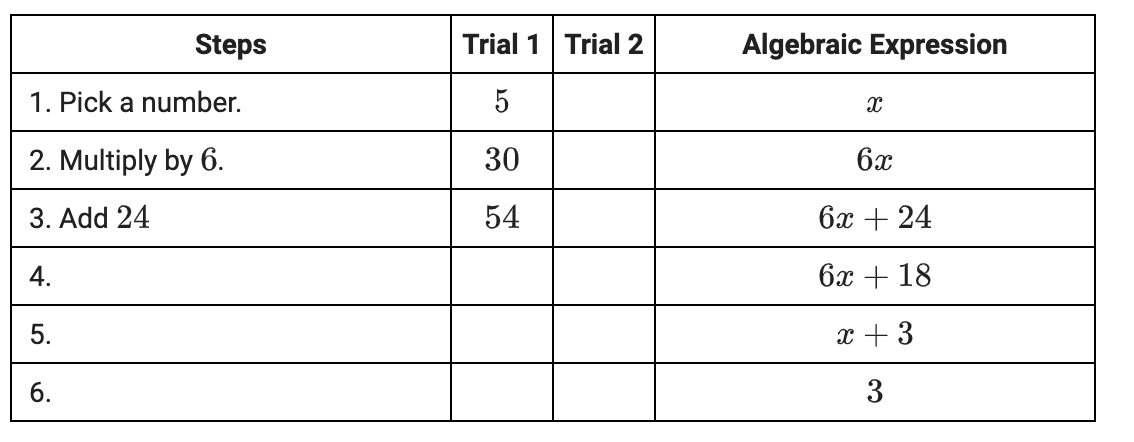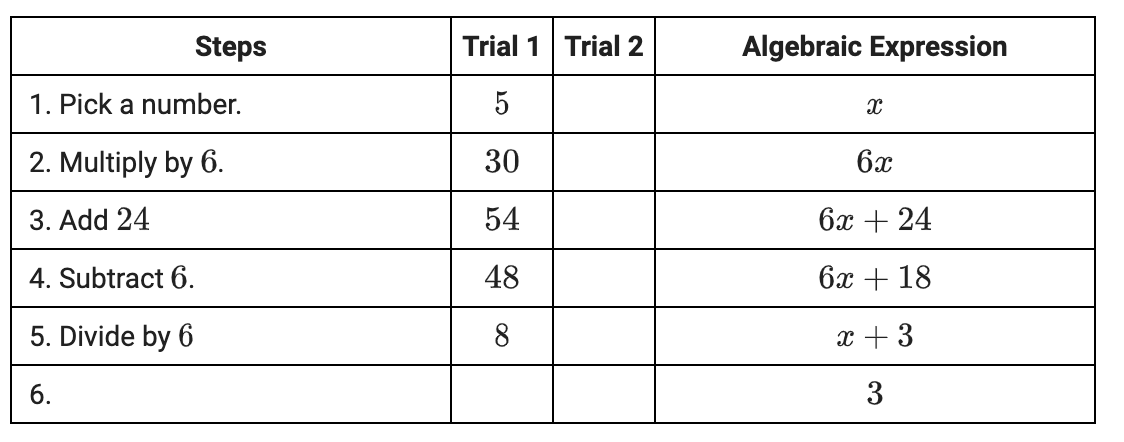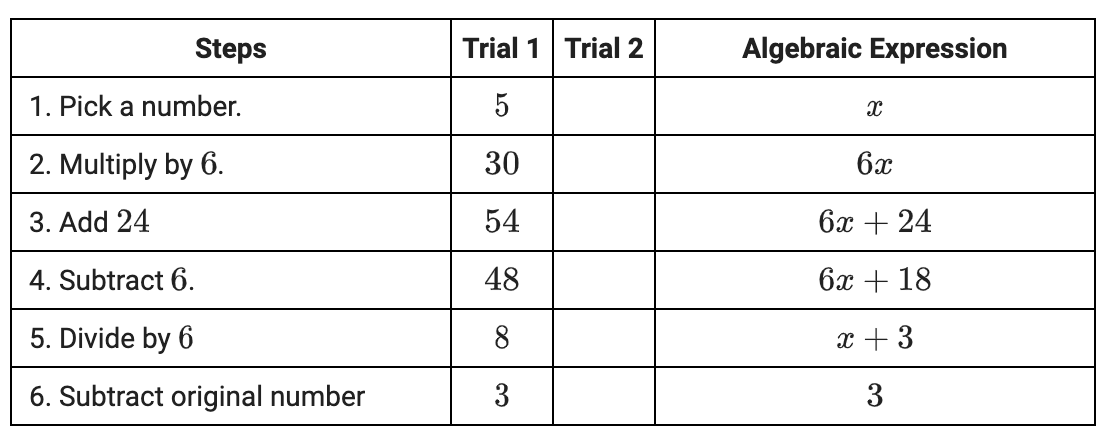Now, go back and pick a different number for the 2nd trial.